74
Computational Geometry - I
Computational Geometry
codemonk
tutorial

This article has been written with the combined efforts of Ayush Jaggi and Arjit. There are a lot of parts for the Computational Geometry articles. This is just a slight introduction for all of you!

Geometry is a branch of mathematics concerned with questions of shape, size, relative position of figures, and the properties of space. It is a branch of computer science devoted to the study of algorithms which can be stated in terms of geometry.

So where is computational geometry used? What are the real world scenarios where we use these concepts. Think about video games where scenes have to be displayed containing environment as a player moves around. To take care of what a particular user is seeing in what direction, how will he move around when an action happens and a lot of other things are taken care of using computational geometry. A data structure known as a binary space partition is commonly used for this purpose.

Almost all common geometry problems are about two-dimensional Euclidean geometry. Three-(or more-)dimensional problems are pretty rare. This is because the time complexity of an algorithm often grows quickly with the number of dimensions.

If we look at the problems involving computational geometry, they are interesting theoretically and often involve a lot of proofs, intuitions, corollaries etc. A lot of people have the common notion that knowing a couple of problems involving geometry and not understanding how things are happening will be enough - for a while, it might just be enough, but in the long run, you might start falling short of things.

"For example, consider the following situation: You own an art gallery and want to install security cameras to guard our artwork. But we’re under a tight budget, so we want to use as few cameras as possible. How many cameras do we need? This is commonly known as the Art Gallery problem.

When we translate this to computational geometric notation, the ‘floor plan’ of the gallery is just a simple polygon. And with some elbow grease, we can prove that n/3 cameras are always sufficient for a polygon on n vertices, no matter how messy it is. The proof itself uses dual graphs, some graph theory, triangulations, and more. Here, we see a clever proof technique and a result that is curious enough to be appreciated on its own. But if theoretical relevance isn’t enough for you…"

• A Point.
A Point (or vector) is defined by its coordinates- x, y, z. In case of 2-D problems, z = 0.

A point can be represented by a struct in C as follows:

typedef struct {
int x, y, z;
} Point; // set z = 0 for a 2D point


• Vector:
What is a vector? A vector is one of the simplest structures which is used when one is dealing with a geometry problem. A vector has a direction and a magnitude. In case of two dimensions, a vector is represented as a pair of numbers: x and y, which gives both a direction and a magnitude.

Vectors are commonly used to represent directed line segments. Representation of a vector is usually in its component-ized form, where its projections along the major axes are used as quantities by which it is defined.

In three dimensions:

v = vxi + vyj + vzk

There are multiple mathematical operations that can be performed on vectors. The simplest of these is addition. Adding two vectors will result in a new vector. If you have two vectors (x1, y1) and (x2, y2), then, the sum of the two vectors is simply (x1+x2, y1+y2). It doesn’t matter which order you add them up in – just like regular addition.

• Dot Product:
The dot product is a quantity which is the length of one vector times the length of the amount of another vector parallel to that vector. Given two vectors u and v, the dot product of the two vectors would be: |u||v|cos (θ). θ is the angle between the two vectors u and v. |u| is called the norm of the vector, and in a 2-D geometry problem is simply the length of the vector, √(x2+y2). From this, one can see that if two vectors are perpendicular, the dot product would be 0. The dot product is greatest when the lines are parallel. We can take dot products of vectors with any number of elements.

So, the point to note? Adding two vectors and multiplying a vector by a scalar is done component-wise.

• Cross Product:

The cross product of two 2-D vectors is (x1y2 - y1x2).

The cross product takes two vectors and produces a vector that is perpendicular to both vectors.
Like the dot product, A x B = |A||B|Sin(θ). |θ| is the angle between the two vectors, but θ is negative or positive based on the right-hand rule. It means that if A is less than 180 degrees clockwise from B, the value is positive. It also means that cross-product of two parallel vectors is zero.The cross product is also NOT commutative: u x v = -v x u.

Fun fact: Absolute value of |A||B|Sin(θ) is equal to the area of the parallelogram with two of its sides formed by A and B.

The cross product in three dimensions is given by: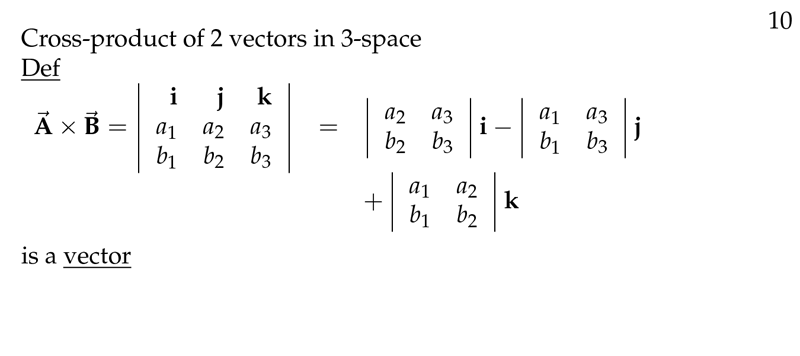It can be remembered as the determinant of the matrix.

Code:

def dot(A, B, C):
AB, BC  = *2, *2
AB = B  - A;
AB = B - A;
BC = C - B;
BC = C - B;
return (AB * BC + AB * BC)

def cross(A, B, C):
AB, BC  = *2, *2
AB = B  - A;
AB = B - A;
AC = C - A;
AC = C - A;
return (AB * AC + AB * AC)


• CCW:

One of the most important operation that is essential to many geometric solutions is the counter-clockwise-function. Given the three coordinates of 3 points, this function returns 1 if the points are in counter-clockwise order, returns -1, if they are in clockwise order and returns 0 if they are collinear.

def ccw(a, b, c):
dx1 = b - a;
dx2 = c - a;
dy1 = b - a;
dy2 = c - a;
if dx1*dy2 > dy1*dx2;
return 1;
if dx1*dy2 < dy1*dx2;
return -1;
return 0;


• Line.
The primal way to specify a line L is by giving two distinct points, P0 and P1, on it. Generally, we have the following applications relating to lines:

• Distance of a Point to an Infinite Line.
Suppose we are given an infinite line L, and any point P. Let d denote the shortest distance from P to L. As we all know, this is the length of a perpendicular dropped from P to L.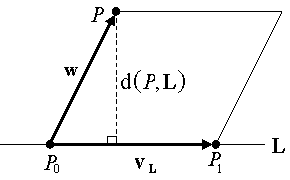The distance d is given by: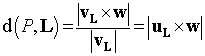Here, uL= unit direction vector of L.

For a 2-point Line, the formula can be written as:A line segment: is a part of a line that is bounded by two distinct end points, and contains every point on the line between its end points. A closed line segment includes both endpoints, while an open line segment excludes both endpoints; a half-open line segment includes exactly one of the endpoints.

A finite segment S consists of the points of a line that are between two endpoints P0 and P1. Note that P's perpendicular base is actually outside the segment's range. In this case, we must determine which end of the segment is closest to P.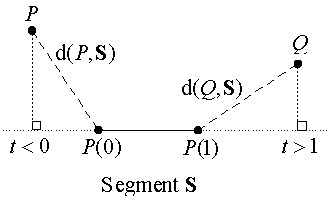An easy way to do this is to consider the angles between the segment P0-P1 and the vectors from each endpoint to the point P. We basically check whether the angle is acute or obtuse. These conditions are easily tested by computing the dot product of the vectors involved and testing whether it is positive, negative, or zero.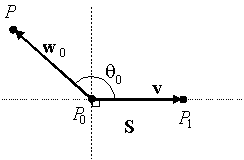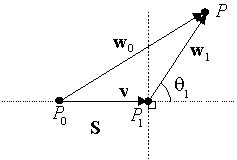• Polygon:

Polygons are two-dimensional shapes. They are made of straight lines, and the shape is "closed". If all angles are equal and all sides are equal, then it is regular, otherwise it is irregular.

The general formula of area of a polygon:

Area = 1/2 * Σ (From i=0 to n-1) Xi * Yi+1 - Xi+1 + Yi

It returns us the area of any polygon, given its vertices, where n is the number of vertices.

Final code:

#include<bits/stdc++.h>
using namespace std;

/*
Assume that we already have the classes for the following objects:
Point and Vector with coordinates {float x, y, z;} (z=0  for 2D)
and appropriate operators for:
Point  = Point ± Vector
Vector = Point - Point
Vector = Scalar * Vector
Line with defining endpoints {Point P0, P1;}
Segment with defining endpoints {Point P0, P1;}
Plane with a point and a normal vector {Point V0; Vector  n;}
*/

// dot product (3D) which allows vector operations in arguments
#define dot(u,v)   ((u).x * (v).x + (u).y * (v).y + (u).z * (v).z)
#define norm(v)     sqrt(dot(v,v))     // norm = length of  vector
#define d(u,v)      norm(u-v)          // distance = norm of difference

//===================================================================
/*
dist_Point_to_Line(): get the distance of a point to a line
Input:  a Point P and a Line L (in any dimension)
Return: the shortest distance from P to L
*/
float dist_Point_to_Line( Point P, Line L)
{
Vector v = L.P1 - L.P0;
Vector w = P - L.P0;
double c1 = dot(w,v);
double c2 = dot(v,v);
double b = c1 / c2;
Point Pb = L.P0 + b * v;
return d(P, Pb);
}
//===================================================================
/*
dist_Point_to_Segment(): get the distance of a point to a segment
Input:  a Point P and a Segment S (in any dimension)
Return: the shortest distance from P to S
*/
float dist_Point_to_Segment( Point P, Segment S)
{
Vector v = S.P1 - S.P0;
Vector w = P - S.P0;

double c1 = dot(w,v);
if ( c1 <= 0 )
return d(P, S.P0);

double c2 = dot(v,v);
if ( c2 <= c1 )
return d(P, S.P1);

double b = c1 / c2;
Point Pb = S.P0 + b * v;
return d(P, Pb);
}
//===================================================================
/* dist_Point_to_Plane(): get distance (and perp base) from a point to a plane
Input:  P  = a 3D point
PL = a  plane with point V0 and normal n
Output: *B = base point on PL of perpendicular from P
Return: the distance from P to the plane PL
*/
float dist_Point_to_Plane( Point P, Plane PL, Point* B)
{
float    sb, sn, sd;

sn = -dot( PL.n, (P - PL.V0));
sd = dot(PL.n, PL.n);
sb = sn / sd;

*B = P + sb * PL.n;
return d(P, *B);
}
//===================================================================


Some problems you guys can try solving:
1. https://www.hackerearth.com/problem/algorithm/aldrin-justice/description/
2. https://www.hackerearth.com/problem/algorithm/good-points/description/

Take a look at some common geometry formulas you might need.
For having fun in Python with Computational Geometry, read this.
For more such applications of computational geometry, take a look at these lecture notes.